# Lorene::Dyn_eos_poly Class Reference [Equations of state]

Polytropic equation of state (relativistic case) for use in dynamical code. More...

#include <dyneos.h>

Inheritance diagram for Lorene::Dyn_eos_poly: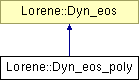List of all members.

## Public Member Functions

Dyn_eos_poly (double gamma, double kappa)
Standard constructor (sets both m_0 and mu_0 to 1).
Dyn_eos_poly (double gamma, double kappa, double mass)
Standard constructor with individual particle mass (sets mu_0 to 1).
Dyn_eos_poly (double gamma, double kappa, double mass, double mu_zero)
Standard constructor with individual particle mass and zero-pressure chemical potential.
Dyn_eos_poly (const Dyn_eos_poly &)
Copy constructor.
virtual ~Dyn_eos_poly ()
Destructor.
void operator= (const Dyn_eos_poly &)
Assignment to another Dyn_eos_poly.
virtual bool operator== (const Dyn_eos &) const
Comparison operator (egality).
virtual bool operator!= (const Dyn_eos &) const
Comparison operator (difference).
virtual int identify () const
Returns a number to identify the sub-classe of Dyn_eos the object belongs to.
double get_gam () const
Returns the adiabatic index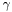(cf. Eq. (3)).
double get_kap () const
Returns the pressure coefficient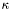(cf.
double get_m_0 () const
Return the individual particule mass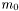(cf.
double get_mu_0 () const
Return the relativistic chemical potential at zero pressure [unit: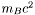, with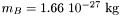].
virtual void sauve (FILE *) const
Save in a file.
virtual double ent_nbar_p (double nbar, const Param *par=0x0) const
Computes the log-enthalpy from the baryon density and extra parameters (virtual function implemented in the derived classes).
virtual double ener_nbar_p (double nbar, const Param *par=0x0) const
Computes the total energy density from the baryon density and extra parameters (virtual function implemented in the derived classes).
virtual double press_nbar_p (double nbar, const Param *par=0x0) const
Computes the pressure from the baryon density and extra parameters (virtual function implemented in the derived classes).
virtual double csound_nbar_p (double nbar, const Param *par=0x0) const
Computes the sound speed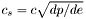from the baryon density with extra parameters (virtual function implemented in the derived classes).
const string & get_name () const
Returns the EOS name.
void set_name (const string &)
Sets the EOS name.
virtual bool operator== (const Dyn_eos &) const =0
Comparison operator (egality).
virtual bool operator!= (const Dyn_eos &) const =0
Comparison operator (difference).
Scalar ent_nbar (const Scalar &nbar, int nzet, int l_min=0, Param *par=0x0) const
Computes the log-enthalpy field from the baryon density field and extra parameters.
Scalar ener_nbar (const Scalar &nbar, int nzet, int l_min=0, Param *par=0x0) const
Computes the total energy density from the baryon density and extra parameters.
Scalar press_nbar (const Scalar &nbar, int nzet, int l_min=0, Param *par=0x0) const
Computes the pressure from the baryon density and extra parameters.
Scalar csound_nbar (const Scalar &nbar, int nzet, int l_min=0, Param *par=0x0) const
Computes the sound speedfrom the baryon density with extra parameters.

## Static Public Member Functions

static Dyn_eoseos_from_file (FILE *)
Construction of an EOS from a binary file.
static Dyn_eoseos_from_file (ifstream &)
Construction of an EOS from a formatted file.

## Protected Member Functions

Dyn_eos_poly (FILE *)
Constructor from a binary file (created by the function sauve(FILE*) ).
Dyn_eos_poly (ifstream &)
Constructor from a formatted file.
void set_auxiliary ()
Computes the auxiliary quantities gam1 , unsgam1 , gam1sgamkap from the values of gam and kap.
virtual ostream & operator>> (ostream &) const
Operator >>.
void calcule (const Scalar &thermo, int nzet, int l_min, double(Dyn_eos::*fait)(double, const Param *) const, Param *par, Scalar &resu) const
General computational method for Scalar 's.

## Protected Attributes

double gam
Adiabatic index(cf. Eq. (3)).
double kap
Pressure coefficient(cf.
double m_0
Individual particule mass(cf.
double mu_0
Relativistic chemical potential at zero pressure [unit:, with].
double gam1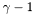double kapsgam1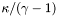double gamkapsgam1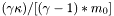double rel_mu_0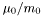string name
EOS name.

## Friends

Dyn_eosDyn_eos::eos_from_file (FILE *)
The construction functions from a file.
Dyn_eosDyn_eos::eos_from_file (ifstream &)
ostream & operator<< (ostream &, const Dyn_eos &)
Display.

## Detailed Description

Polytropic equation of state (relativistic case) for use in dynamical code.

This equation of state (EOS) corresponds to identical relativistic particles of rest mass is, whose total energy density e is related to their numerical density n by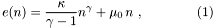where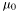is the chemical potential at zero pressure. The relativistic (i.e. including rest mass energy) chemical potential is then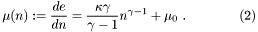The pressure is given by the (zero-temperature) First Law of Thermodynamics: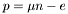, so thatThe log-enthalpy is defined as the logarithm of the ratio of the enthalpy par particle by the partical rest mass energy :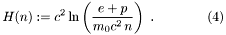According to the (zero-temperature) First Law of Thermodynamics, the log-enthalpy is related to the chemical potential by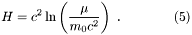()

Definition at line 366 of file dyneos.h.

## Constructor & Destructor Documentation

 Lorene::Dyn_eos_poly::Dyn_eos_poly ( double gamma, double kappa )

Standard constructor (sets both m_0 and mu_0 to 1).

The individual particle massis set to the mean baryon mass.

Parameters:
 gamma adiabatic index(cf. Eq. (3)) kappa pressure coefficient(cf. Eq. (3)) [unit: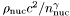], where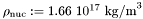and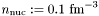Definition at line 61 of file dyneos_poly.C.

References set_auxiliary().

 Lorene::Dyn_eos_poly::Dyn_eos_poly ( double gamma, double kappa, double mass )

Standard constructor with individual particle mass (sets mu_0 to 1).

Parameters:
 gamma adiabatic index(cf. Eq. (3)) kappa pressure coefficient(cf. Eq. (3)) [unit:], whereandmass individual particule mass(cf. Eq. (1) [unit:]

Definition at line 70 of file dyneos_poly.C.

References set_auxiliary().

 Lorene::Dyn_eos_poly::Dyn_eos_poly ( double gamma, double kappa, double mass, double mu_zero )

Standard constructor with individual particle mass and zero-pressure chemical potential.

Parameters:
 gamma adiabatic index(cf. Eq. (3)) kappa pressure coefficient(cf. Eq. (3)) [unit:], whereandmass individual particule mass(cf. Eq. (1)) [unit:] mu_zero Relativistic chemical potential at zero pressure [unit:, with]. (standard value: 1)

Definition at line 79 of file dyneos_poly.C.

References set_auxiliary().

 Lorene::Dyn_eos_poly::Dyn_eos_poly ( const Dyn_eos_poly & eosi )

Copy constructor.

Definition at line 88 of file dyneos_poly.C.

References set_auxiliary().

 Lorene::Dyn_eos_poly::Dyn_eos_poly ( FILE * fich )  [protected]

Constructor from a binary file (created by the function sauve(FILE*) ).

This constructor is protected because any EOS construction from a binary file must be done via the function Dyn_eos::eos_from_file(FILE*) .

Definition at line 98 of file dyneos_poly.C.

References Lorene::fread_be(), gam, kap, m_0, mu_0, and set_auxiliary().

 Lorene::Dyn_eos_poly::Dyn_eos_poly ( ifstream & fich )  [protected]

Constructor from a formatted file.

This constructor is protected because any EOS construction from a formatted file must be done via the function Dyn_eos::eos_from_file(ifstream&) .

Definition at line 119 of file dyneos_poly.C.

References gam, kap, m_0, mu_0, and set_auxiliary().

 Lorene::Dyn_eos_poly::~Dyn_eos_poly ( )  [virtual]

Destructor.

Definition at line 140 of file dyneos_poly.C.

## Member Function Documentation

 void Lorene::Dyn_eos::calcule ( const Scalar & thermo, int nzet, int l_min, double(Dyn_eos::*)(double, const Param *) const fait, Param * par, Scalar & resu ) const [protected, inherited]

General computational method for Scalar 's.

Parameters:
 thermo [input] thermodynamical quantity (for instance the density field) from which the thermodynamical quantity resu is to be computed. nzet [input] number of domains where resu is to be computed. l_min [input] index of the innermost domain is which resu is to be computed [default value: 0]; resu is computed only in domains whose indices are in [l_min,l_min+nzet-1] . In the other domains, it is set to zero. fait [input] pointer on the member function of class Dyn_eos which performs the pointwise calculation. par possible extra parameters of the EOS resu [output] result of the computation.

Definition at line 129 of file dyneos.C.

 Scalar Lorene::Dyn_eos::csound_nbar ( const Scalar & nbar, int nzet, int l_min = 0, Param * par = 0x0 ) const [inherited]

Computes the sound speedfrom the baryon density with extra parameters.

Parameters:
 nbar [input, unit:] baryon density nzet number of domains where the derivative dln(e)/dln(H) is to be computed. l_min index of the innermost domain is which the coefficient dln(n)/dln(H) is to be computed [default value: 0]; the derivative dln(e)/dln(H) is computed only in domains whose indices are in [l_min,l_min+nzet-1] . In the other domains, it is set to zero. par possible extra parameters of the EOS
Returns: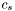[unit: c]

Definition at line 233 of file dyneos.C.

 double Lorene::Dyn_eos_poly::csound_nbar_p ( double nbar, const Param * par = 0x0 ) const [virtual]

Computes the sound speedfrom the baryon density with extra parameters (virtual function implemented in the derived classes).

Parameters:
 nbar [input, unit:] baryon density par possible extra parameters of the EOS
Returns:[unit: c]

Implements Lorene::Dyn_eos.

Definition at line 312 of file dyneos_poly.C.

References gam, gam1, kapsgam1, mu_0, Lorene::pow(), and Lorene::sqrt().

 Scalar Lorene::Dyn_eos::ener_nbar ( const Scalar & nbar, int nzet, int l_min = 0, Param * par = 0x0 ) const [inherited]

Computes the total energy density from the baryon density and extra parameters.

Parameters:
 nbar [input, unit:] baryon density nzet number of domains where the energy density is to be computed. l_min index of the innermost domain is which the energy density is to be computed [default value: 0]; the energy density is computed only in domains whose indices are in [l_min,l_min+nzet-1] . In the other domains, it is set to zero. par possible extra parameters of the EOS
Returns:
energy density [unit: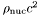], whereDefinition at line 209 of file dyneos.C.

 double Lorene::Dyn_eos_poly::ener_nbar_p ( double nbar, const Param * par = 0x0 ) const [virtual]

Computes the total energy density from the baryon density and extra parameters (virtual function implemented in the derived classes).

Parameters:
 nbar [input, unit:] baryon density par possible extra parameters of the EOS
Returns:
energy density e [unit:], whereImplements Lorene::Dyn_eos.

Definition at line 292 of file dyneos_poly.C.

References gam, kapsgam1, mu_0, and Lorene::pow().

 Scalar Lorene::Dyn_eos::ent_nbar ( const Scalar & nbar, int nzet, int l_min = 0, Param * par = 0x0 ) const [inherited]

Computes the log-enthalpy field from the baryon density field and extra parameters.

Parameters:
 nbar [input, unit:] baryon density nzet number of domains where the baryon density is to be computed. l_min index of the innermost domain is which the baryon density is to be computed [default value: 0]; the baryon density is computed only in domains whose indices are in [l_min,l_min+nzet-1] . In the other domains, it is set to zero. par possible extra parameters of the EOS
Returns:
ent log-enthalpy H defined by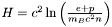, where e is the (total) energy density, p the pressure, n the baryon density, and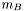the baryon mass.

Definition at line 196 of file dyneos.C.

 double Lorene::Dyn_eos_poly::ent_nbar_p ( double nbar, const Param * par = 0x0 ) const [virtual]

Computes the log-enthalpy from the baryon density and extra parameters (virtual function implemented in the derived classes).

Parameters:
 nbar [input, unit:] baryon density par possible extra parameters of the EOS
Returns:
ent log-enthalpy H defined by, where e is the (total) energy density, p the pressure, n the baryon density, andthe baryon mass.

Implements Lorene::Dyn_eos.

Definition at line 282 of file dyneos_poly.C.

References gam1, gamkapsgam1, Lorene::log(), Lorene::pow(), and rel_mu_0.

 Dyn_eos * Lorene::Dyn_eos::eos_from_file ( ifstream & fich )  [static, inherited]

Construction of an EOS from a formatted file.

The fist line of the file must start by the EOS number, according to the following conventions (same as fo the classes Eos ):

• 1 = relativistic polytropic EOS (class Dyn_eos_poly ).
• 2 = Newtonian polytropic EOS (class Dyn_eos_poly_newt ).
• 17 = Tabulated EOS (class Dyn_eos_tab ).
• 20 = Consistent EOS from table (class Dyn_eos_cons ).

The second line in the file should contain a name given by the user to the EOS. The following lines should contain the EOS parameters (one parameter per line), in the same order than in the class declaration.

Definition at line 295 of file dyneos.C.

 Dyn_eos * Lorene::Dyn_eos::eos_from_file ( FILE * fich )  [static, inherited]

Construction of an EOS from a binary file.

The file must have been created by the function sauve(FILE*) .

Definition at line 256 of file dyneos.C.

 double Lorene::Dyn_eos_poly::get_gam ( ) const

Returns the adiabatic index(cf. Eq. (3)).

Definition at line 172 of file dyneos_poly.C.

References gam.

 double Lorene::Dyn_eos_poly::get_kap ( ) const

Returns the pressure coefficient(cf.

Eq. (3)) [unit:], whereand.

Definition at line 177 of file dyneos_poly.C.

References kap.

 double Lorene::Dyn_eos_poly::get_m_0 ( ) const

Return the individual particule mass(cf.

Eq. (1)) [unit:].

Definition at line 182 of file dyneos_poly.C.

References m_0.

 double Lorene::Dyn_eos_poly::get_mu_0 ( ) const

Return the relativistic chemical potential at zero pressure [unit:, with].

Definition at line 187 of file dyneos_poly.C.

References mu_0.

 const string & Lorene::Dyn_eos::get_name ( ) const [inherited]

Returns the EOS name.

Definition at line 103 of file dyneos.C.

References Lorene::Dyn_eos::name.

 int Lorene::Dyn_eos_poly::identify ( ) const [virtual]

Returns a number to identify the sub-classe of Dyn_eos the object belongs to.

Implements Lorene::Dyn_eos.

Definition at line 246 of file dyneos.C.

 virtual bool Lorene::Dyn_eos::operator!= ( const Dyn_eos & ) const [pure virtual, inherited]

Comparison operator (difference).

 bool Lorene::Dyn_eos_poly::operator!= ( const Dyn_eos & eos_i ) const [virtual]

Comparison operator (difference).

Definition at line 242 of file dyneos_poly.C.

References operator==().

 void Lorene::Dyn_eos_poly::operator= ( const Dyn_eos_poly & eosi )

Assignment to another Dyn_eos_poly.

Definition at line 146 of file dyneos_poly.C.

References gam, kap, m_0, mu_0, Lorene::Dyn_eos::name, set_auxiliary(), and Lorene::Dyn_eos::set_name().

 virtual bool Lorene::Dyn_eos::operator== ( const Dyn_eos & ) const [pure virtual, inherited]

Comparison operator (egality).

 bool Lorene::Dyn_eos_poly::operator== ( const Dyn_eos & eos_i ) const [virtual]

Comparison operator (egality).

Definition at line 198 of file dyneos_poly.C.

References gam, identify(), Lorene::Dyn_eos::identify(), kap, m_0, and mu_0.

 ostream & Lorene::Dyn_eos_poly::operator>> ( ostream & ost ) const [protected, virtual]

Operator >>.

Implements Lorene::Dyn_eos.

Definition at line 263 of file dyneos_poly.C.

References gam, kap, m_0, and mu_0.

 Scalar Lorene::Dyn_eos::press_nbar ( const Scalar & nbar, int nzet, int l_min = 0, Param * par = 0x0 ) const [inherited]

Computes the pressure from the baryon density and extra parameters.

Parameters:
 nbar [input, unit:] baryon density nzet number of domains where the pressure is to be computed. l_min index of the innermost domain is which the pressure is to be computed [default value: 0]; the pressure is computed only in domains whose indices are in [l_min,l_min+nzet-1] . In the other domains, it is set to zero. par possible extra parameters of the EOS
Returns:
pressure [unit:], whereDefinition at line 221 of file dyneos.C.

 double Lorene::Dyn_eos_poly::press_nbar_p ( double nbar, const Param * par = 0x0 ) const [virtual]

Computes the pressure from the baryon density and extra parameters (virtual function implemented in the derived classes).

Parameters:
 nbar [input, unit:] baryon density par possible extra parameters of the EOS
Returns:
pressure p [unit:], whereImplements Lorene::Dyn_eos.

Definition at line 302 of file dyneos_poly.C.

References gam, kap, and Lorene::pow().

 void Lorene::Dyn_eos_poly::sauve ( FILE * fich ) const [virtual]

Save in a file.

Reimplemented from Lorene::Dyn_eos.

Definition at line 252 of file dyneos_poly.C.

References Lorene::fwrite_be(), gam, kap, m_0, and mu_0.

 void Lorene::Dyn_eos_poly::set_auxiliary ( )  [protected]

Computes the auxiliary quantities gam1 , unsgam1 , gam1sgamkap from the values of gam and kap.

Definition at line 164 of file dyneos_poly.C.

References gam, gam1, gamkapsgam1, kap, kapsgam1, m_0, mu_0, and rel_mu_0.

 void Lorene::Dyn_eos::set_name ( const string & name_i )  [inherited]

Sets the EOS name.

Definition at line 98 of file dyneos.C.

References Lorene::Dyn_eos::name.

## Friends And Related Function Documentation

 Dyn_eos* Dyn_eos::eos_from_file ( FILE * )  [friend]

The construction functions from a file.

 ostream& operator<< ( ostream & , const Dyn_eos & )  [friend, inherited]

Display.

## Member Data Documentation

 double Lorene::Dyn_eos_poly::gam [protected]

Adiabatic index(cf. Eq. (3)).

Definition at line 373 of file dyneos.h.

 double Lorene::Dyn_eos_poly::gam1 [protected]Definition at line 395 of file dyneos.h.

 double Lorene::Dyn_eos_poly::gamkapsgam1 [protected]Definition at line 397 of file dyneos.h.

 double Lorene::Dyn_eos_poly::kap [protected]

Pressure coefficient(cf.

Eq. (3)) [unit:], whereand.

Definition at line 380 of file dyneos.h.

 double Lorene::Dyn_eos_poly::kapsgam1 [protected]Definition at line 396 of file dyneos.h.

 double Lorene::Dyn_eos_poly::m_0 [protected]

Individual particule mass(cf.

Eq. (1)) [unit:].

Definition at line 385 of file dyneos.h.

 double Lorene::Dyn_eos_poly::mu_0 [protected]

Relativistic chemical potential at zero pressure [unit:, with].

(standard value: 1)

Definition at line 391 of file dyneos.h.

 string Lorene::Dyn_eos::name [protected, inherited]

EOS name.

Definition at line 74 of file dyneos.h.

 double Lorene::Dyn_eos_poly::rel_mu_0 [protected]Definition at line 398 of file dyneos.h.

The documentation for this class was generated from the following files:

Generated on 7 Dec 2019 for LORENE by1.6.1